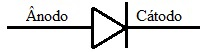# Semiconductor diode

The semiconductor diode is an electronic device made of silicon or germanium whose function is to transform alternating current into direct current.

The semiconductor diode is an electronic device made of silicon or germanium that has the function of rectifying the electric current or switching a circuit. It is used in electronic devices such as television, computer, stereo, among others. The symbol used for diodes in diagrams is as follows:The diode is symbolized by an arrow that indicates the direction of the electric current.

How a semiconductor diode works

The current supplied by energy companies is alternating, that is, they change their polarity between positive and negative with a frequency of 60 Hz. However, most electronic devices that we use only work with direct current, that is, only one polarity. We say that the diode works as a closed switch (zero resistance) for one polarity of the input voltage and as an open switch (infinite resistance) for the opposite polarity.

Therefore, the function of the diode in a circuit is to let the electric current pass in only one polarity. Look at the figure:

The graph shows the diode input voltage oscillating between positive and negative

After passing through the diode, the voltage has only one polarity:

The conduction of electric current will depend on how the diode is biased, which can be in two ways:

• Forward polarization: In this type of polarization the positive pole of the voltage source is connected to the P side of the diode. This makes the positive side even more positive and the N side even more negative. Electric charges are able to cross the potential barrier between the P side and the N side of the diode, therefore, current conduction;
• Reverse Bias: The positive terminal of the voltage source is connected to the N side of the PN junction of the diode. This causes the potential barrier to increase. In this case, the resistance of the circuit is too high, and the electric current cannot pass through it.

Check Also
Close